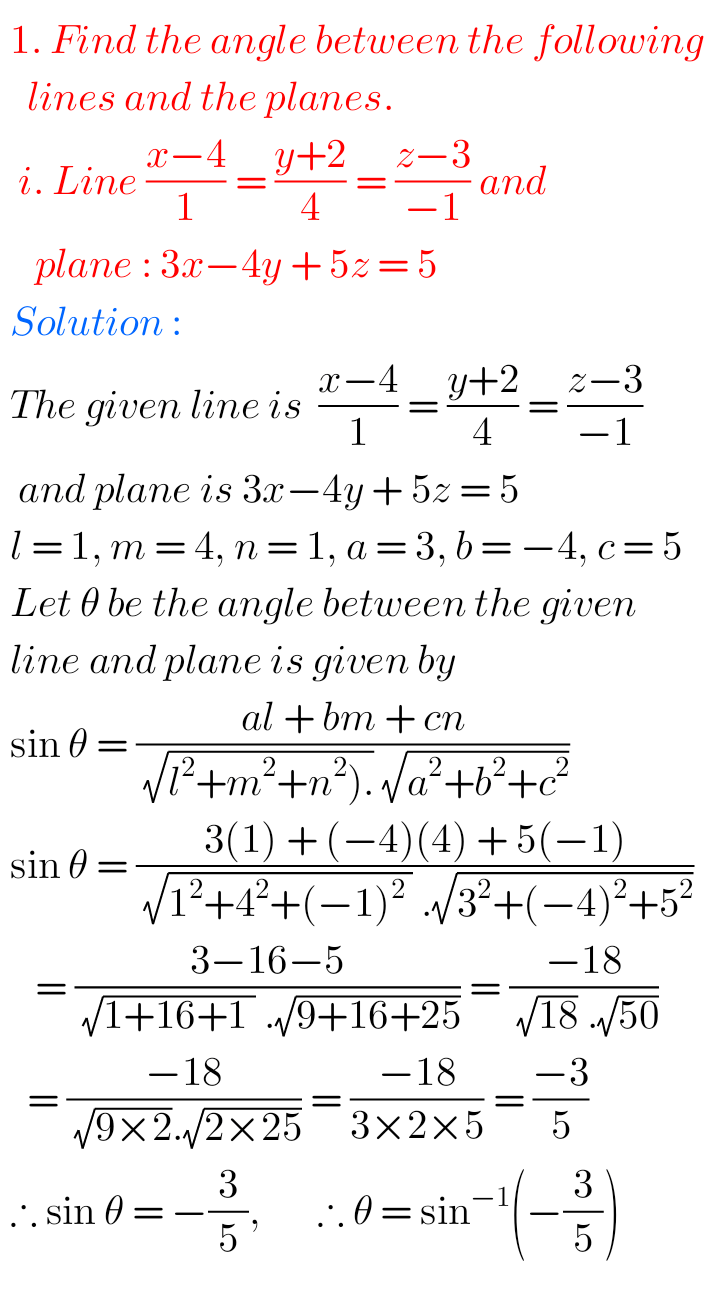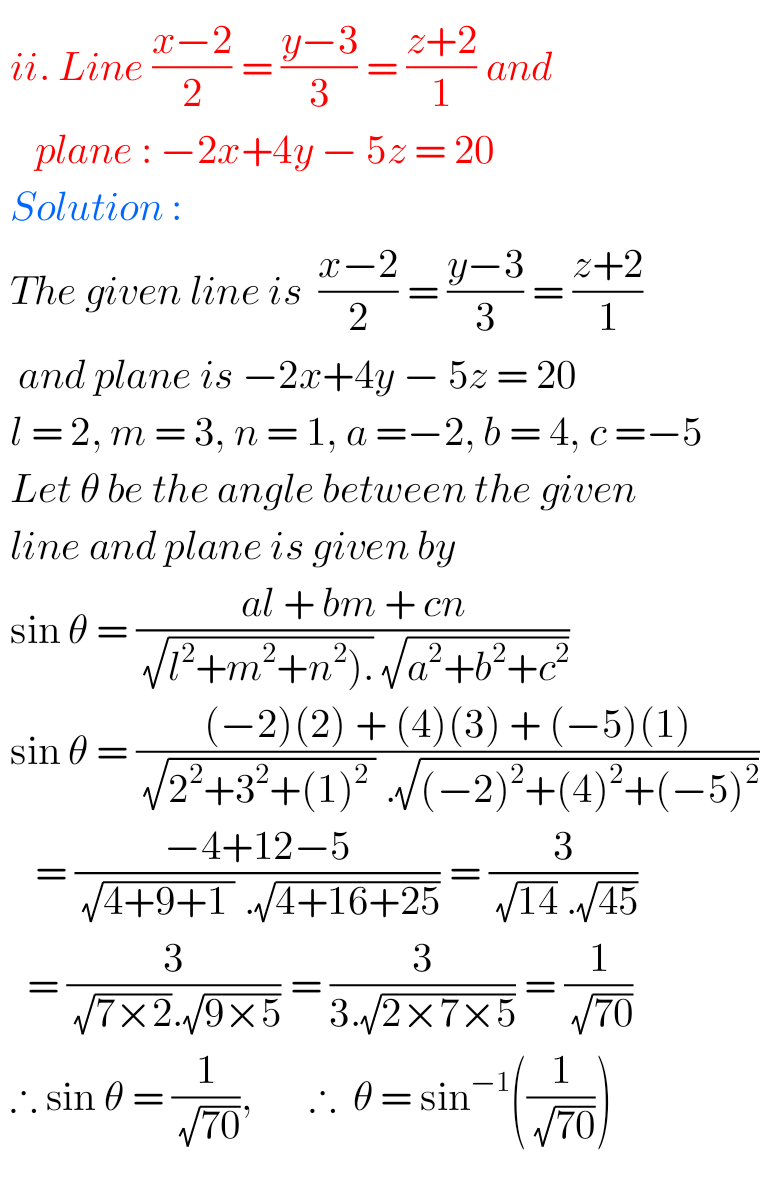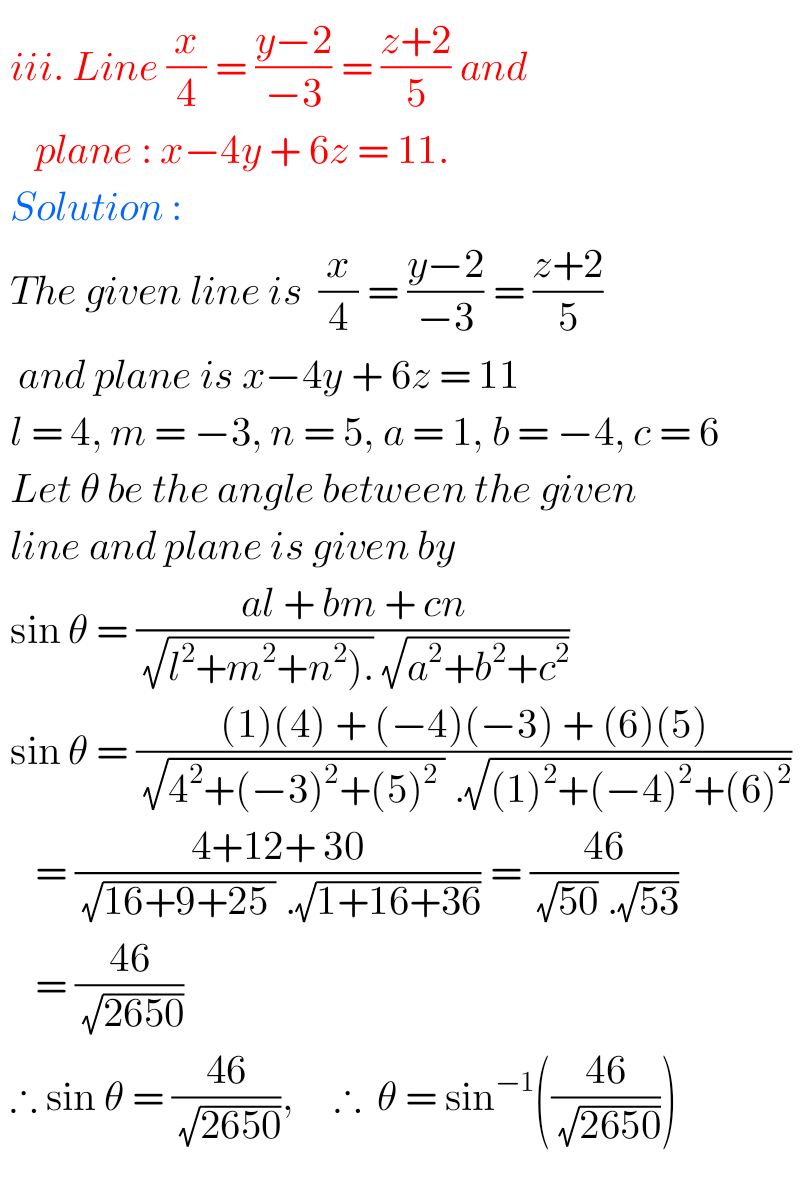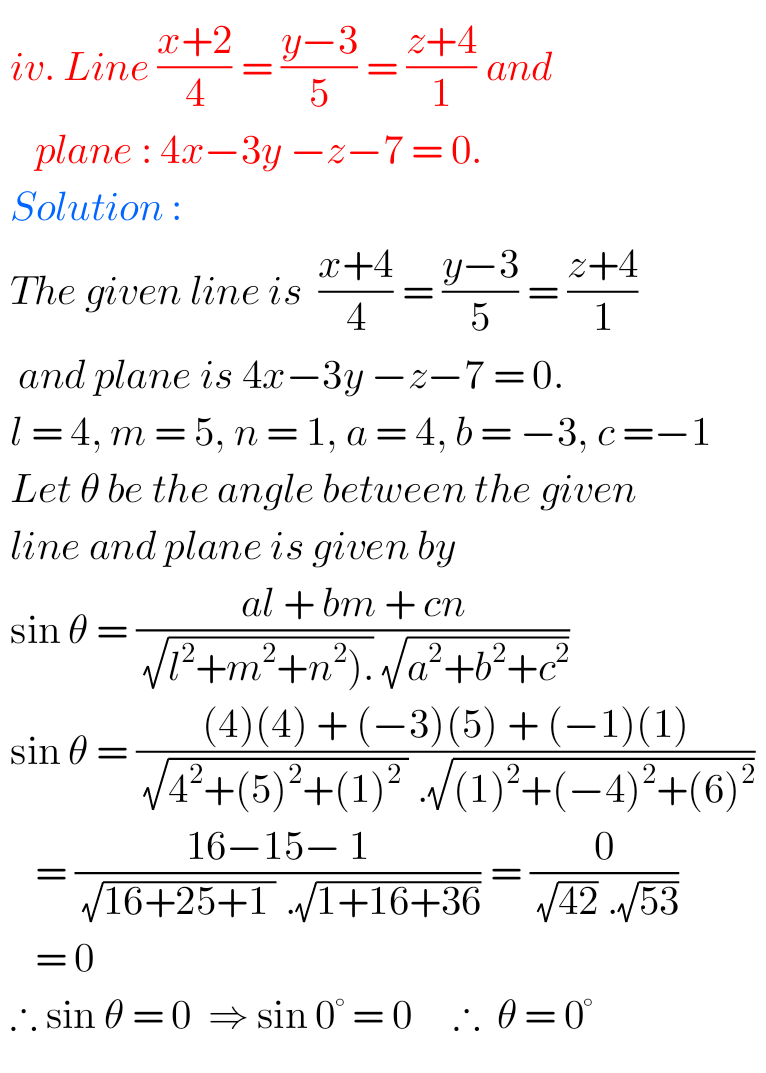# Straight line check your progress 36.4 solutions NIOS

NIOS Mathematics 311 book 2 Module IX Chapter 36 check your progress 36.4 solutions are given.

You should study the textbook lesson Straight Line very well.

You should also observe and practice all problems and solutions given in the textbook.

Observe the given solutions and try them in your own method.

You can also see.

NIOS Maths 311 book 2 solutions for some chapters

Staight Line

Terminal exercise chapter 36

## Maths 311 book 2 chapter 36 check your progress 36.4 solutions NIOS

Straight LIne

Chapter 36

1. Find the angle between the following lines and the planes. i. line (x-4)/1 = (y + 2)/4 = (z -3)/-1 and plane 3x -4y + 5z = 5ii. line (x – 2)/2 = (y -3)/3 = (z + 2)/1 and -2x + 4y – 5z = 20### NIOS maths Straight Line solutions check your progress 36.4

iii. Line x/4 = (y -2)/-3 = (z + 2)/5 and plane: x -4y + 6z = 11iv. Line (x + 2)/4 = (y -3)/5 = (z + 4)/1Note: Observe the solutions and try them in your own method.

SSC maths class 10 solutions

Ncert maths class 6 solutions

NIOS maths 311 book 2 solutions for some chapters

NCERT class 7th maths solutions